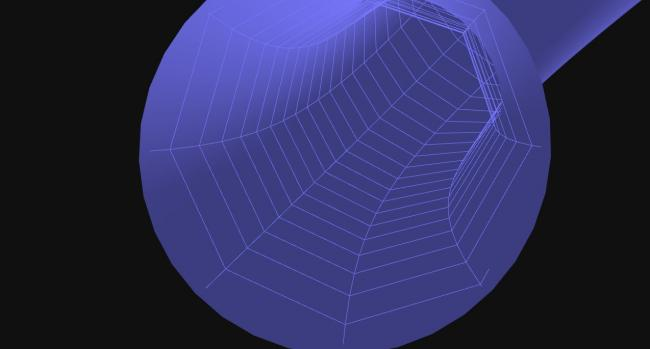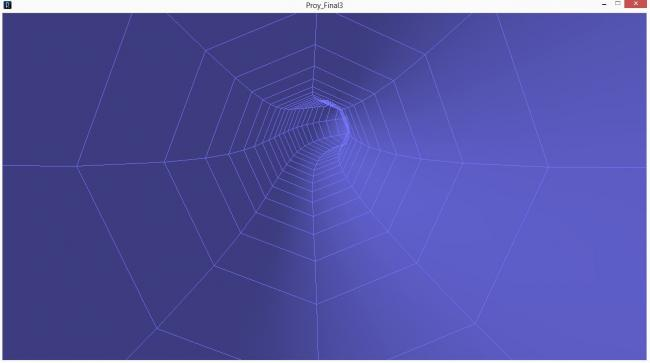#### Howdy, Stranger!

We are about to switch to a new forum software. Until then we have removed the registration on this forum.

# Shapes 3D library camera doesn´t focus whole composition

edited July 2018

Hi guys, well i´ve been using the Shapes3D library, y downloaded a sketch for a tunnel and used the examples from the library, this example had 2 java codes added, it showed me the tunnel full at the display but didn´t let me add images, use the displayWidth and displayHeight commands or use present ir at full screen, so i took out the java codes added, now it works perfectly, but doesn´t get the whole tunel just the center of it, like if the camera had smaller vision o was far from the composition. I´ve tried everything, closing up the camera, scaling, making bigger the tunnel, nothing works.

`````` /* OpenProcessing Tweak of *@*http://www.openprocessing.org/sketch/25597*@* */
/* !do not delete the line above, required for linking your tweak if you upload again */
import shapes3d.utils.*;
import shapes3d.animation.*;
import shapes3d.*;

int enviar=0;

// Path dependent variables
I_PathGen[] path;
int nbrPaths = 1;
int currPath = 0;
float[] speedFactor;

// The tubes
PathTube atube, wtube;
int tubeType = 0;
int nbrTubeTypes = 2;
final int SLICES = 300, SEGMENTS = 8;

// The shuttle
//Toroid train;
Box[] bcar;
final int nbrBoxCars = 0;

// Used for train/car orientation to the tube
final PVector FORWARD_AXIS = new PVector(0, 1, 0);
final PVector LEFT_AXIS = new PVector(-1, 0, 0);
final PVector RIGHT_AXIS = new PVector(0, 0, -1);
PVector normal, tangent, position;
PVector camPos, camLookAt, camUp;
Rot rot;
float[] ang;

// SHUTTLE SPEED CONTROL VARIABLES
float speed = 0.06, pathFactor = 1, elapsed;
float paraT = 0.5;  // starting position on curve
float camParaT;
long st, ct;      // keep track of running time

void setup() {

size(1280, 690, P3D);
// Create the path generator
//  path = new Tube (this,4,6);
path = new I_PathGen[nbrPaths];

// hacer una clase para definir el parámetro
path = new Lissajous(1, 1, 1);
speedFactor = new float[nbrPaths];

// Create the tube
atube = new PathTube(this, path[currPath], 40, SLICES, 35, false);
atube.drawMode(Shape3D.SOLID);
atube.visible(false, Shape3D.BOTH_CAP);

// Create path cage
wtube = new PathTube(this, path[currPath], 38, (SLICES/2), 8, true);
wtube.stroke(color(120, 120, 255));
wtube.strokeWeight(1);
wtube.drawMode(Shape3D.WIRE); // estructura alámbrica
wtube.visible(false, Shape3D.BOTH_CAP);

// Initialise spacing and speed factors
float l = atube.length(400);
speedFactor[currPath] = 800 / l;

// Create the box cars
bcar = new Box[nbrBoxCars];
for (int i = 0; i < nbrBoxCars; i++) {
bcar[i] = new Box(this, 0);
}
// Initialise times
st = ct = millis();
}

void draw() {
// scale(10,10,10);
background(16);
lights();
// Used to get frame-rate independent speed
ct = millis();
//image(img, 0, 0);
elapsed = (ct - st)/1000.0;
st = ct;
paraT += elapsed * speed * speedFactor[currPath];
paraT = (paraT > 1) ? paraT-1 : paraT;
normal = atube.getNormal(paraT);
tangent = atube.getTangent(paraT);
position = atube.getPoint(paraT);

// Calculate camera position to move with the shuttle
normal.normalize();
camUp = PVector.mult(normal, -1);
println (camLookAt);
camParaT = paraT - 0.0;
camParaT = (camParaT > 1) ? camParaT: camParaT;
camPos = atube.getPoint(camParaT);

camLookAt = PVector.mult(tangent, 90);

camera(camPos.x, camPos.y, camPos.z,
camLookAt.x, camLookAt.y, camLookAt.z,
camUp.x, camUp.y, camUp.z);

float bparaT = paraT;
for (int i = 0; i < nbrBoxCars; i++) {
bparaT = (bparaT < 0) ? bparaT + 1 : bparaT;
normal = atube.getNormal(bparaT);
position = atube.getPoint(bparaT);
rot = new Rot(FORWARD_AXIS, RIGHT_AXIS, tangent, normal);
ang = rot.getAngles(RotOrder.XYZ);
bcar[i].moveTo(position);
bcar[i].rotateTo(ang);
bcar[i].draw();
}
wtube.draw();
atube.draw();
}

void keyReleased() {
if (key >= '0' && key <= '9') {
speed = (key - 48) / 50.0;
}
}
``````Tagged:

• All the Shape3D examples you see in my portfolio on Open Processing were created with Processing 1.5.1 and when you download them you also get the Processing core library (core.jar) and Shapes3D library (shapes3d.jar) from the time the applet was uploaded. In other words old versions. It means that the P3D renderer used is the 1.5.1 non-OpenGL version instead of the OpenGL version used in V2+

This sketch (like most of my library demos in my OP portfolio) is included in the examples that come with the library so I recommend you use that rather than the download.

Looking at the 2 pictures you posted the problem is caused because objects close to the view point are not draw. This is called the near zone this is easily fixed simple change the default perspective in setup with

``````void setup() {

size(1280, 690, P3D);
float fov = PI/3.0;
float cameraZ = (height/2.0) / tan(fov/2.0);
perspective(fov, float(width)/float(height), cameraZ/100.0, cameraZ*10.0);
//      near zone default is cameraZ/10.0 changed to ^^^^^
``````

Not sure what you mean by

but didn´t let me add images

do you mean textures for the tube?

I have no solution for the displayWidth / displayHeight / present, sorry about that.

• the "loadImage" function was disabled, actually what i need is how locate my camera in the picture 1, because this code i posted doesn´t hace any bugs anymore, but you can only see the tunnel in the middle of the screen which mess up my concept.

• the "loadImage" function was disabled

That is part of Processing and not part of Shapes3D.

but you can only see the tunnel in the middle of the screen

You have to move the camPos outside the tube. Delete the code I suggested in my last post and try changing line 102 to

`camPos.add(PVector.mult(camUp, 40));`

which mess up my concept.

• ``````path = new Lissajous(1, 1, 1);